Question

The integrated rate laws for zero-, first-, and second-order reaction may be arranged such that they resemble the equation for a straight line, y=mx+by=mx+b.

 Order Integrated Rate Law Graph Slope 0 [A]=−kt+[A]0[A]=−kt+[A]0 [A] vs. t[A] vs. t −k 1 ln[A]=−kt+ln[A]0ln⁡[A]=−kt+ln⁡[A]0 ln[A] vs. tln[A] vs. t −k 2 1[A]= kt+1[A]01[A]= kt+1[A]0 1[A] vs. t1[A] vs. t k

Part A

The reactant concentration in a zero-order reaction was 8.00×10−2 MM after 130 ss and 4.00×10−2 MM after 380 ss . What is the rate constant for this reaction?

Express your answer with the appropriate units. Indicate the multiplication of units, as necessary, explicitly either with a multiplication dot or a dash.

Part C

The reactant concentration in a first-order reaction was 8.00×10−2 MM after 25.0 ss and 4.90×10−3 MM after 60.0 ss . What is the rate constant for this reaction?

Express your answer with the appropriate units. Indicate the multiplication of units, as necessary, explicitly either with a multiplication dot or a dash.

Part D

The reactant concentration in a second-order reaction was 0.460 MM after 300 ss and 3.60×10−2 MM after 705 ss . What is the rate constant for this reaction?

Express your answer with the appropriate units. Indicate the multiplication of units, as necessary, explicitly either with a multiplication dot or a dash.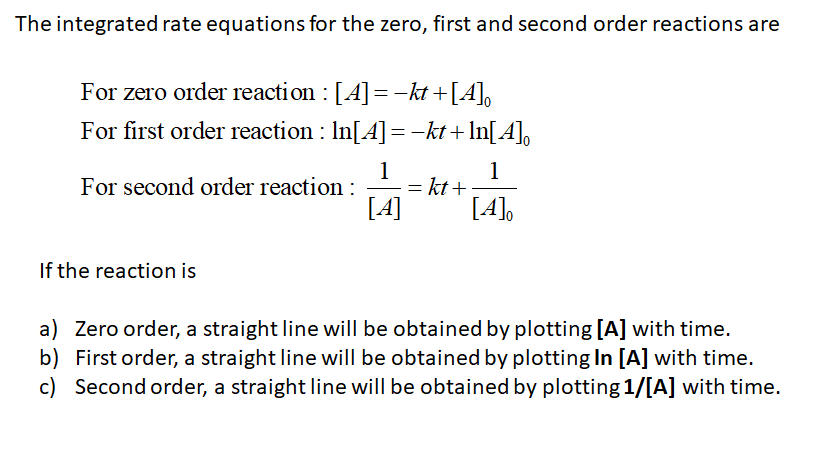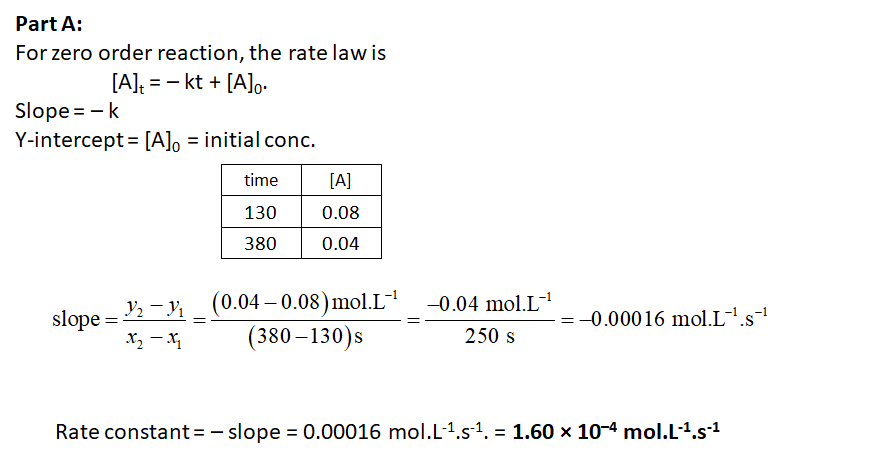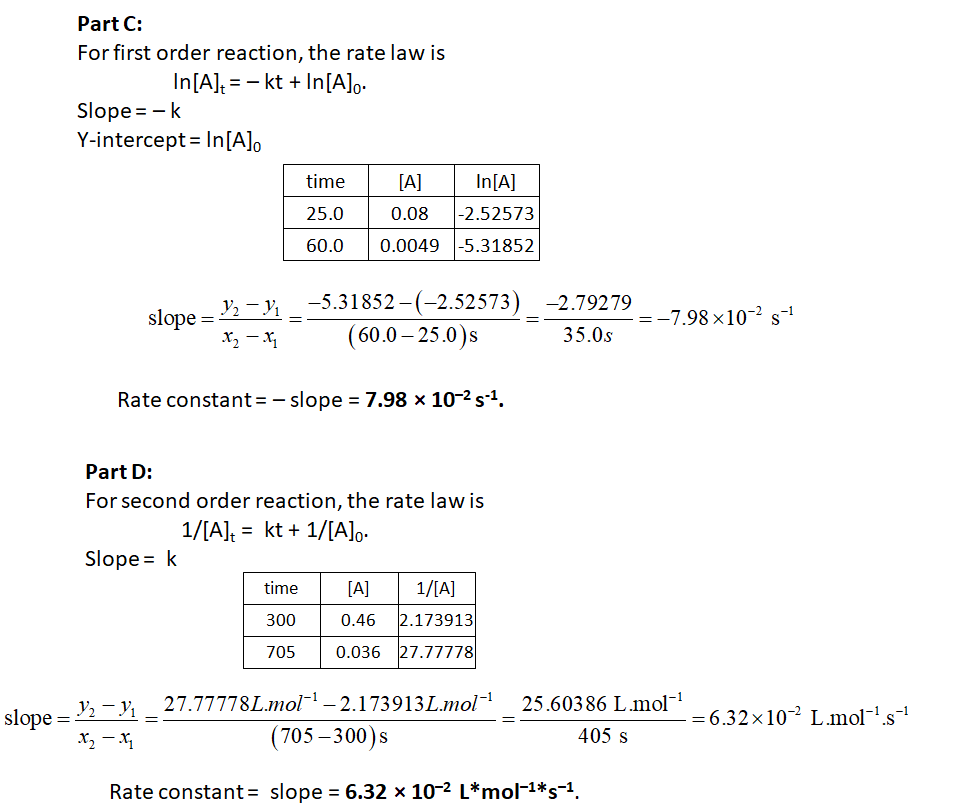#### Earn Coins

Coins can be redeemed for fabulous gifts.

Similar Homework Help Questions
• ### + Using Integrated Rate Laws The integrated rate laws for zero-, first-, and second-order reaction may...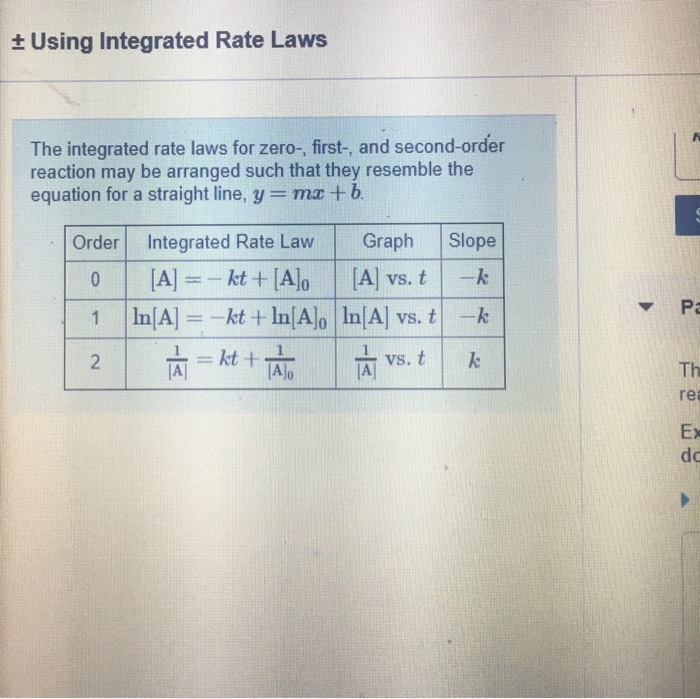+ Using Integrated Rate Laws The integrated rate laws for zero-, first-, and second-order reaction may be arranged such that they resemble the equation for a straight line, y = mx + b. Slope Order O 1 2 Integrated Rate Law Graph [A] = - kt + [A] [A] vs. t In[A] = -kt + In[A], In[A] vs. t LÀ=kt + TA LÀ vs. t -k Review Constants Periodic Table Part A The reactant concentration in a zero-order reaction was...

• ### + Using Integrated Rate Laws The integrated rate laws for zero-, first-, and second-order reaction may be arrang...+ Using Integrated Rate Laws The integrated rate laws for zero-, first-, and second-order reaction may be arranged such that they resemble the equation for a straight line, y = mx + b. Slope Order O 1 2 Integrated Rate Law Graph [A] = - kt + [A] [A] vs. t In[A] = -kt + In[A], In[A] vs. t LÀ=kt + TA LÀ vs. t -k Review Constants Periodic Table Part A The reactant concentration in a zero-order reaction was...

• ### ± Using Integrated Rate Laws Part A The reactant concentration in a zero-order reaction The integrated...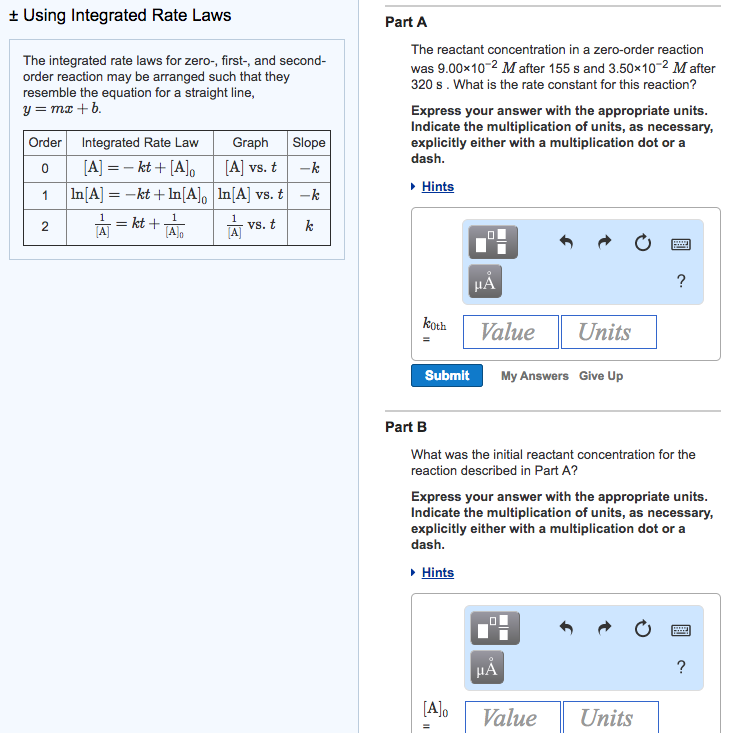± Using Integrated Rate Laws Part A The reactant concentration in a zero-order reaction The integrated rate laws for zero-, first-, and second order reaction may be arranged such that they resemble the equation for a straight line y=mx + b was 9.00x102 M after 155 s and 3.50x102 M after 320 s. What is the rate constant for this reaction? Express your answer with the appropriate units Indicate the multiplication of units, as necessary explicitly either with a multiplication...

• ### ing Integrated Rate Laws < 10 of 11 > Review Constants Periodic Table ne integrated rate...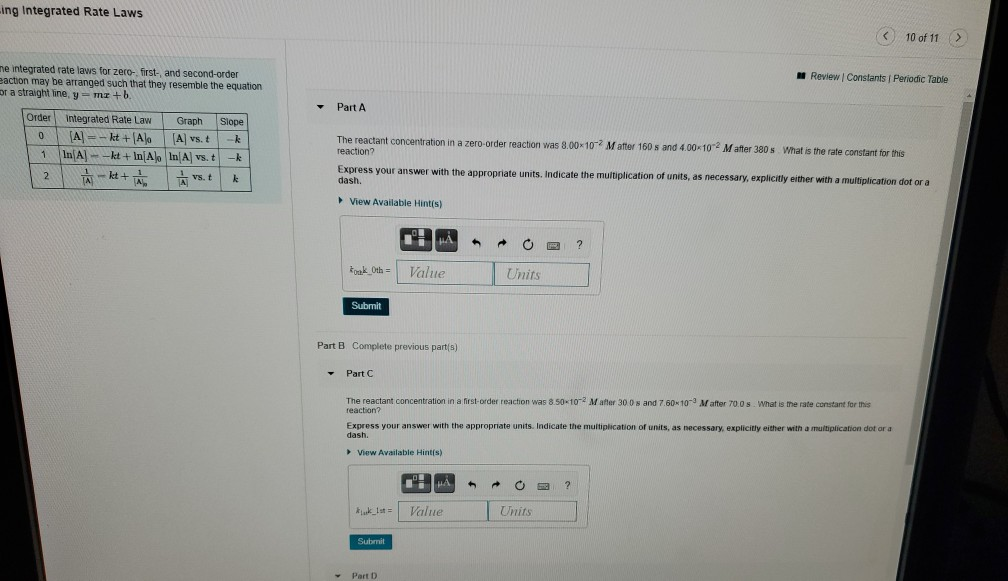ing Integrated Rate Laws < 10 of 11 > Review Constants Periodic Table ne integrated rate laws for zero, first- and second-order eaction may be arranged such that they resemble the equation or a straight line, ymr + b Part A Order 0 Integrated Rate Law Graph Slope [A] = - kt +(Alo (A) vs. t -k In A-kt+In Al In A vs. t -k Avst k 1 The reactant concentration in a zero order reaction was 8.00-10-2 Mafter 160...

• ### 3 of 19 ReviewConelants1 Pariodc Table The integrated rate laws for zero, Srst, and second-order meaction...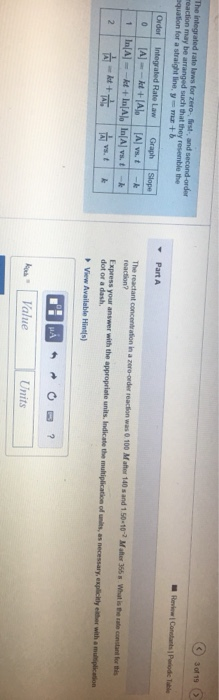3 of 19 ReviewConelants1 Pariodc Table The integrated rate laws for zero, Srst, and second-order meaction may be arranged such that they resemble the aquation for a straight line, sy me+b Part A Order Integrated Rate Law Graph Slope A t+A In A)-kt+In A In A) vs. t The reactant concentration in a zero-order reaction was 0 100 Maher 140s and 1.50-10 reaction? A vs. -A Malter 355 What is the ate contant for is 1 -k Express your answer...

• ### us Course Home Homework Chapter 14 Using Integrated Rate Laws 8 of 16 > The integrated...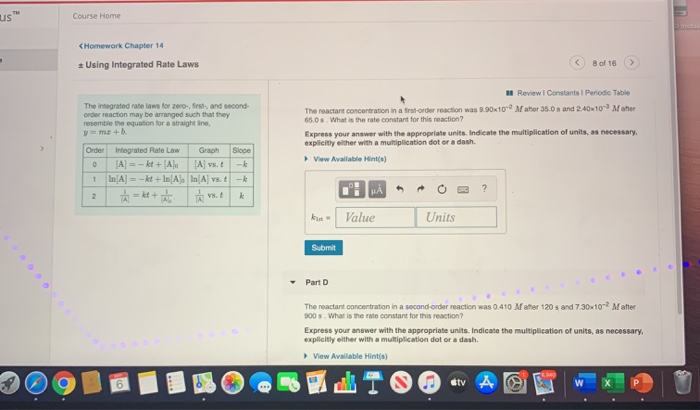us Course Home Homework Chapter 14 Using Integrated Rate Laws 8 of 16 > The integrated rate laws for zero-, f and second- order reaction may be arranged such that they resemble the equation for a straight line y = m + b a Review Constants Periodic Table The reactant concentration in a first-order reaction was 9.90x10-2 Mator 35.0 and 240x10-3 Matter 65.0 s What is the rate constant for this reaction? Express your answer with the appropriate units, Indicate...

• ### HW 4 ± Using Integrated Rate Laws Resources previous | 1 of 11 | next» ±...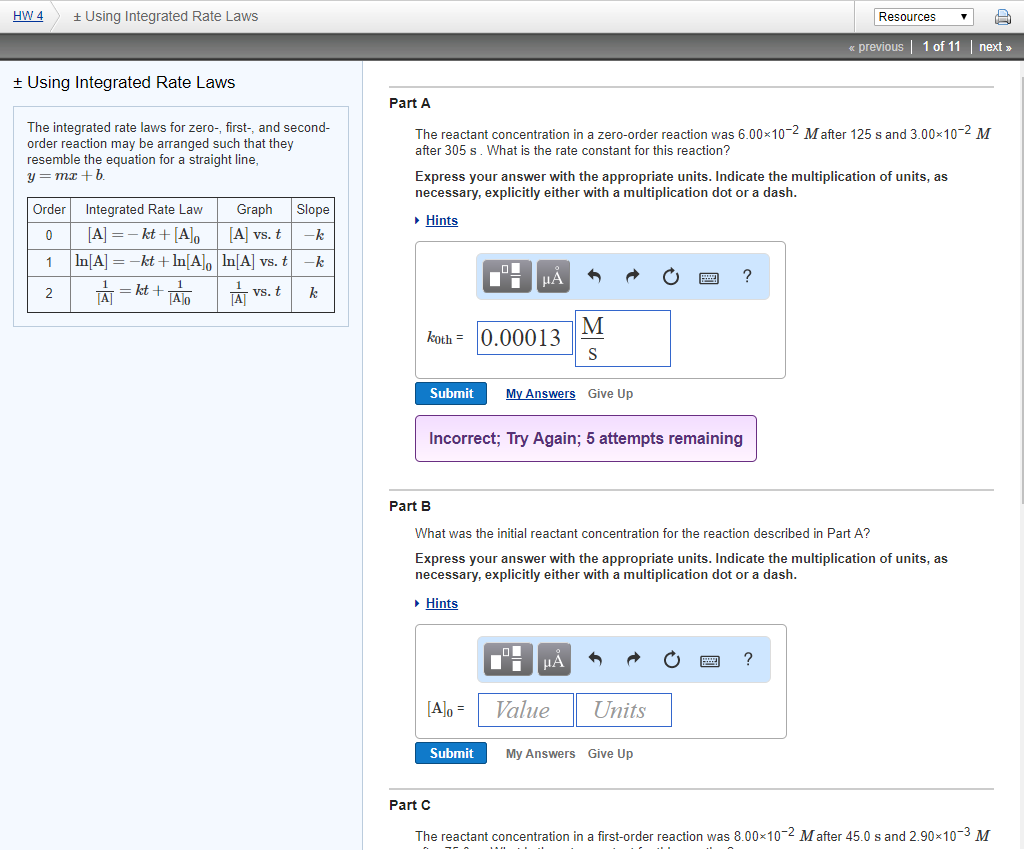HW 4 ± Using Integrated Rate Laws Resources previous | 1 of 11 | next» ± Using Integrated Rate Laws Part A The integrated rate laws for zero-, first-, and second- order reaction may be arranged such that they resemble the equation for a straight line y=mx + b Mafter 125 s and 3.00x10 M The reactant concentration in a zero-order reaction was 6.00x10 after 305 s. What is the rate constant for this reaction? Express your answer with the...

• ### Part A The reactant concentration in a zero-order reaction was 6.00×10?2M after 105 s and 4.00×10?2M...

Part A The reactant concentration in a zero-order reaction was 6.00×10?2M after 105 s and 4.00×10?2M after 335 s . What is the rate constant for this reaction? Express your answer with the appropriate units. Indicate the multiplication of units, as necessary, explicitly either with a multiplication dot or a dash. Part B What was the initial reactant concentration for the reaction described in Part A? Express your answer with the appropriate units. Indicate the multiplication of units, as necessary,...

• ### The reactant concentration in a zero-order reaction was 0.100 M after 165 s and 2.50×10−2M after...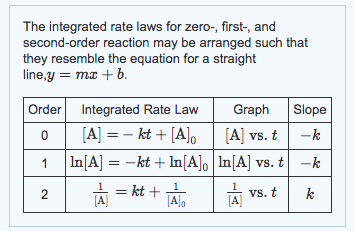The reactant concentration in a zero-order reaction was 0.100 M after 165 s and 2.50×10−2M after 345 s, and the rate constant of the reaction is 4.17*10 What was the initial reactant concentration for the reaction described in Part A? Express your answer with the appropriate units. Indicate the multiplication of units, as necessary, explicitly either with a multiplication dot or a dash. The integrated rate laws for zero-, first-, and second-order may be arranged such that they resemble the...

• ### Part A The reactant concentration in a zero-order reaction was 7.00×10−2M after 105 s and 1.50×10−2Mafter...

Part A The reactant concentration in a zero-order reaction was 7.00×10−2M after 105 s and 1.50×10−2Mafter 345 s . What is the rate constant for this reaction? Express your answer with the appropriate units. Indicate the multiplication of units, as necessary, explicitly either with a multiplication dot or a dash. Part B What was the initial reactant concentration for the reaction described in Part A? Express your answer with the appropriate units. Indicate the multiplication of units, as necessary, explicitly...